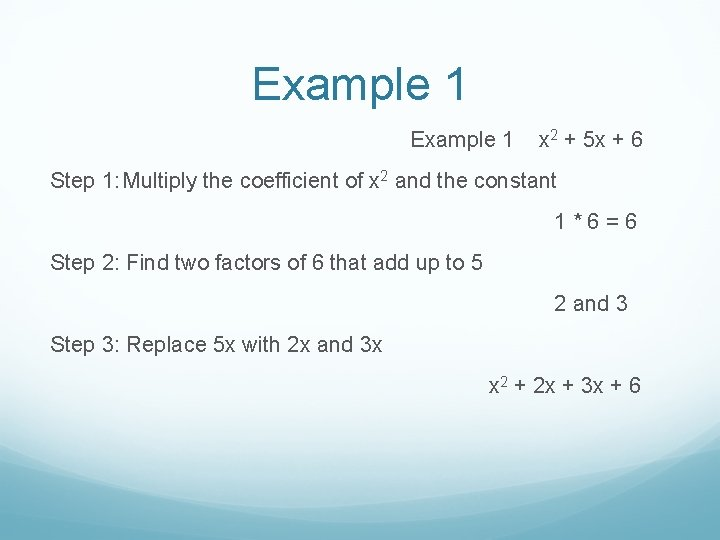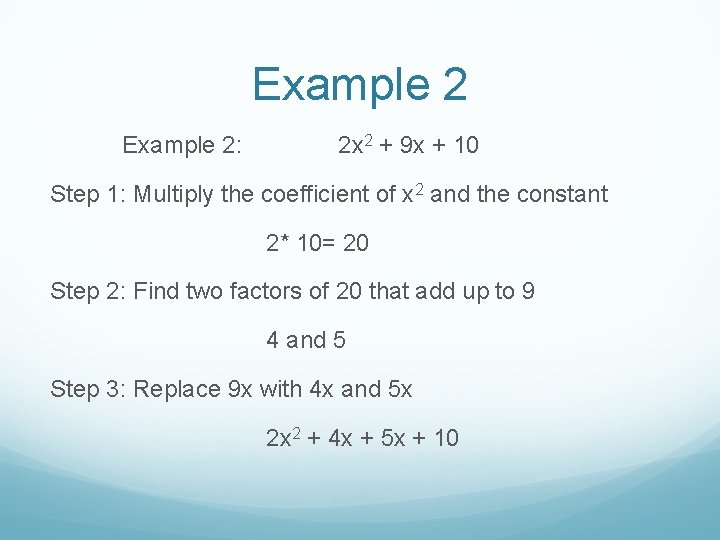# Factoring By Grouping By Brian Chamberlain Brian Fagan

• Slides: 11Factoring By Grouping By, Brian Chamberlain, Brian Fagan, Catherine Perry, and Lauren Jones 2 BFactoring When Factoring a number you break it apart into smaller numbers that can multiplied together to get the original.Factoring By Grouping Group terms with common factors before factoringExample 1 x 2 + 5 x + 6 Step 1: Multiply the coefficient of x 2 and the constant 1*6=6 Step 2: Find two factors of 6 that add up to 5 2 and 3 Step 3: Replace 5 x with 2 x and 3 x x 2 + 2 x + 3 x + 6Example 1 (continued) Step 4: Group first two terms together and group the last two terms together (x 2 + 2 x) + (3 x + 6) Step 5: Factor x(x + 2) + 3(x + 2) Step 6: Factor again (x + 2) (x + 3)Example 2: 2 x 2 + 9 x + 10 Step 1: Multiply the coefficient of x 2 and the constant 2* 10= 20 Step 2: Find two factors of 20 that add up to 9 4 and 5 Step 3: Replace 9 x with 4 x and 5 x 2 x 2 + 4 x + 5 x + 10Example 2 (continued) Step 4: Group first two terms together and group the last two terms together (2 x 2 + 4 x) + (5 x + 10) Step 5: Factor 2 x(x + 2) + 5(x + 2) Step 6: Factor Again (x + 2) (2 x + 5)Example 3 x 2 – 2 x – 35 Step 1: Multiply the coefficient of x 2 and the constant 1 * -35 = -35 Step 2: Find factors of -35 that add up to -2 -7 and 5 Step 3: Replace -2 x with -7 x and 5 x x 2 -7 x + 5 x - 35Example 3 (continued) Step 4: Group first two terms together and group the last two terms together (x 2 - 7 x) + (5 x -35) Step 5: Factor 1 x( x – 7) + 5(x – 7) Step 6: Factor again (x + 5)(x - 7)Example 4: 8 x 2 - 10 x -3 Step 1: Multiply the coefficient of x 2 and the constant 8 * -3 = -24 Step 2: Find factors of -24 that add up to -10 12 and 2 Step 3: Replace -10 x with -12 x and 2 x 8 x 2 + 2 x + -12 x -3Example 4 (continued) Step 4: Group first two terms together and group the last two terms together (8 x 2 + 2 x) + (-12 x – 3) Step 5: Factor 2 x(4 x + 1) + -3(4 x + 1) Step 6: Factor again (4 x + 1) (2 x – 3)